# Why Capacitor and Inductor are used in Filtering Circuit

## Why capacitor and Inductor are used in Filtering Circuit?

In Electrical and electronics circuits the three passive components (Resistor, Capacitor, and Inductor) are playing major role in the history of electricity. Some example: resistor uses as potential divider in may electronics, capacitor and inductor uses as filtering circuit etc. In this article, we are going to study about Why capacitor and inductor are used in Filtering Circuit?.. Lets see

### Why capacitor use as filtering circuit?

Generally, capacitor use to filter voltage spikes, typically instant voltage changes. As you all the types of capacitor is having the property of “Capacitor does not allow the instant voltage changes”.. therefore, capacitor use as voltage filter.

In this, I is the current flow through the capacitor in ampere, C is the value capacitance in Farad and dv/dt is voltage changes.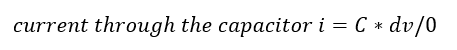In this, instant dv/dt value is nothing but the voltage changes will happen at t=0, therefore…

Anything divided by zero is equal to infinity, hence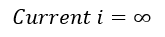Capacitor always try to maintain the source voltage across it, Therefore, whenever the voltage across the capacitor changes instantly, the current through the capacitor reaches maximum. This is practically not possible. therefore, capacitor use as filtering circuit and filter voltage changes (Voltage spikes).

Note: Capacitor does not allow Direct Current, it act as open circuit for DC source and Capacitors are parallel element and it is always connected parallel across the circuit.

Example: SMPS, Amplifier, Signal Transmitter, VFD, Snupper circuit etc.

### Why Inductor use as filtering circuit?

Generally, Inductors are come in the form of chokes and which use to filter Current Transient, typically instant current flow through changes. Inductor is having the property of “Inductor does not allow the instant current changes”.. therefore, inductor use as Current filter. The Voltage across the inductor V is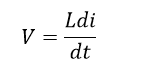In this, V is the Voltage across the inductor in Volts, L is the value Inductance in Henry and di/dt is Current flow through the inductor circuit changes with respect to time t in ampere per sec.

In this, instant di/dt value is nothing but the current changes will happen at t=0, therefore…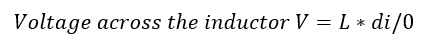Anything divided by zero is equal to infinity, hence the voltage across the inductor V is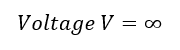Here the voltage across the inductor is maximum, when current through the inductor changes instantly. This is practically not possible. therefore, Inductor use as filtering circuit and filter current changes (current spikes).

Note: Inductor acts as short circuit for Direct Current. Inductor is a current element and it connect series through the circuit. It uses to protect the power electronics devices against switching transient.

Also see: How Inductor choke protect VFD from Current spikes.

Example: SMPS, Amplifier, Signal Transmitter, VFD, Snupper circuit etc.